# Python Machine Learning Client for SAP HANA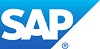Welcome to Python machine learning client for SAP HANA (hana-ml)!

This package enables Python data scientists to access SAP HANA data and build various machine learning models using the data directly in SAP HANA. This page provides an overview of hana-ml.

Python machine learning client for SAP HANA consists of two main parts:

• SAP HANA DataFrame, which provides a set of methods for accessing and querying data in SAP HANA without bringing the data to the client.

• A set of machine learning APIs for developing machine learning models.

Specifically, machine learning APIs are composed of two packages:

• PAL package

PAL package consists of a set of Python algorithms and functions which provide access to machine learning capabilities in SAP HANA Predictive Analysis Library(PAL). SAP HANA PAL functions cover a variety of machine learning algorithms for training a model and then the trained model is used for scoring.

• APL package

Automated Predictive Library (APL) package exposes the data mining capabilities of the Automated Analytics engine in SAP HANA through a set of functions. These functions develop a predictive modeling process that analysts can use to answer simple questions on their customer datasets stored in SAP HANA.

hana-ml uses SAP HANA Python driver (hdbcli) to connect to and access SAP HANA.

A figure of architecture is shown below: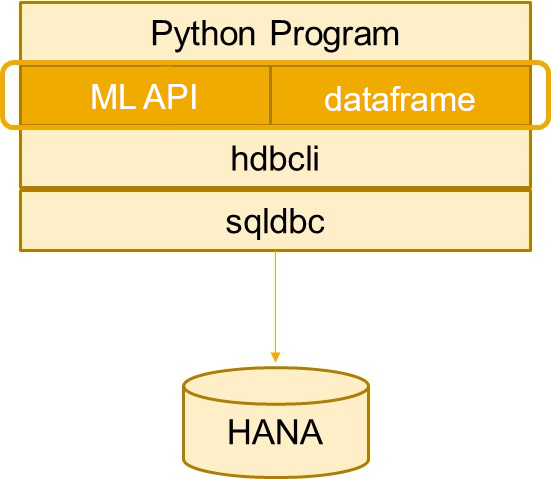## SAP HANA DataFrame

A SAP HANA DataFrame provides a way to view the data stored in SAP HANA without containing any of the physical data. hana-ml makes use of a SAP HANA DataFrame as the input for training and scoring purposes.

A SAP HANA DataFrame hides the underlying SQL statement, providing users with a Python interface to SAP HANA data.

To use a SAP HANA DataFrame, please firstly create a "ConnectionContext" object (a connection to SAP HANA), and then use the methods provided in the library to create a SAP HANA DataFrame. SAP HANA DataFrame is only usable while the connection is open, and is inaccessible once the connection is closed.

The example below shows how to create a "ConnectionContext" object cc and then invoke a method table() to create a simple SAP HANA DataFrame df:

```with ConnectionContext('address', port, 'user', 'password') as cc:
df = (cc.table('MY_TABLE', schema='MY_SCHEMA').filter('COL3 > 5').select('COL1','COL2'))
```

More complex dataframes can also be created by applying a sql() method, for example:

```df = cc.sql('SELECT T.A, T2.B FROM T, T2 WHERE T.C=T2.C')
```

Once df (a SAP HANA DataFrame object) is created, there are several types of functions can be executed on the SAP HANA DataFrame object:

1. DataFrame Manipulation functions: manipulate the rows and columns of data, such as casting columns into new type(cast()), dropping columns(drop()), filling null values(fillna()), joining dataframes(join()), sorting dataframes(sort()), and renaming columns(rename_columns()).

2. Descriptive Functions: statistics relating to the data. For example, showing distinct values(distinct()), and creating dataframes with top n values(head()).

3. DataFrame Transformation functions: such as copy a SAP HANA DataFrame to a Pandas DataFrame(collect()) and upload the data from a Pandas DataFrame into a SAP HANA database and returns a SAP HANA DataFrame(create_dataframe_from_pandas()).

Remember, a SAP HANA DataFrame is a way to view the data stored within SAP HANA, and does not contain any data. If you want to use other Python libraries on the client along with a SAP HANA DataFrame, you need to convert that SAP HANA DataFrame to be a Pandas DataFrame using collect() function. For example, we could convert a SAP HANA DataFrame df to be a Pandas DataFrame as follows.

```pandas_df = df.collect()
```

Following is a sketch of all the available methods for SAP HANA DataFrame:

Methods

 `add_constant`(column_name, value) Adds a new column with constant value. `add_id`([id_col, ref_col, starting_point]) Returns a new SAP HANA DataFrame with a added column. `agg`(agg_list[, group_by]) Returns a SAP HANA DataFrame with the group_by column along with the aggregates. `alias`(alias) Returns a new SAP HANA DataFrame with an alias set. `bin`(col[, strategy, bins, bin_width, bin_column]) Returns a DataFrame with the original columns as well as bin assignments. `cast`(cols[, new_type]) Returns a DataFrame with columns cast to a new type. `collect`([fetch_size, geometries, ...]) Copies the current DataFrame to a new Pandas DataFrame. `concat_columns`(columns, separator) Returns a new DataFrame with splitted column. `corr`(first_col, second_col) Returns a DataFrame that gives the correlation coefficient between two numeric columns. `count`() Computes the number of rows in the SAP HANA DataFrame. `declare_lttab_usage`(usage) Declares whether this DataFrame makes use of local temporary tables. `describe`([cols]) Returns a DataFrame that contains various statistics for the requested column(s). `deselect`(cols) Returns a new DataFrame without columns derived from the current DataFrame. `diff`(index[, periods]) Returns a new SAP HANA DataFrame with differenced values. `disable_validate_columns`() Disable the column validation. `distinct`([cols]) Returns a new SAP HANA DataFrame with distinct values for the specified columns. `drop`(cols) Returns a new SAP HANA DataFrame without the specified columns. `drop_duplicates`([subset]) Returns a new SAP HANA DataFrame with duplicate rows removed. `dropna`([how, thresh, subset]) Returns a new DataFrame with NULLs removed. `dtypes`([subset]) Returns a sequence of tuples describing the DataFrame's SQL types. `empty`() Returns True if this DataFrame has 0 rows. `enable_validate_columns`() Enable the column validation. `fillna`(value[, subset]) Returns a DataFrame with NULLs replaced with a specified value. `filter`(condition) Selects rows that match the given condition. `generate_feature`(targets[, group_by, ...]) Add additional features to the existing dataframe using agg_func and trans_func. `generate_table_type`() Generates a SAP HANA table type based on the dtypes function of the DataFrame. `get_table_structure`() Returns dict format table structure. `has`(col) Returns True if a column is in the DataFrame. `hasna`([cols]) Returns True if a DataFrame contains NULLs. `head`([n]) Returns a DataFrame of the first `n` rows in the current DataFrame. `is_numeric`([cols]) Returns True if the column(s) in the DataFrame are numeric. `join`(other[, condition, how, select]) Returns a new DataFrame that is a join of the current DataFrame with another specified DataFrame. `max`() Gets the maximum value of the columns. `mean`() Gets the mean value of the columns. `median`() Gets the median value of the columns. `min`() Gets the minimum value of the columns. `nullif`(value) Replace certain value with NULL value. `pivot_table`(values, index, columns[, aggfunc]) Returns a DataFrame that gives the pivoted table. `rearrange`([key, features, label, type_ts]) Utility function to generate a new dataframe with [key, features, label] for non time-series dataset and [key, label, features] for time-series dataset. `rename_columns`(names) Returns a DataFrame with renamed columns. `replace`([to_replace, value, regex]) Returns a new DataFrame with replaced value. `save`(where[, table_type, force, ...]) Creates a table or view holding the current DataFrame's data. `save_nativedisktable`(where[, force, save_source]) Materializes dataframe to a SAP HANA native disk. `select`(*cols) Returns a new DataFrame with columns derived from the current DataFrame. `set_index`(keys) Sets the DataFrame using the existing columns. `set_name`(name) Sets the name of the DataFrame. `set_source_table`(table[, schema]) Specifies the source table for the current dataframe. `sort`(cols[, desc]) Returns a new DataFrame sorted by the specified columns. `sort_index`([ascending]) Returns a new DataFrame sorted by the index. `sort_values`(by[, ascending]) Returns a new DataFrame sorted by the specified columns. `split_column`(column, separator, new_column_names) Returns a new DataFrame with splitted column. `stddev`() Gets the stddev value of the columns. `sum`() Gets the summation of the columns. `tail`([n, ref_col]) Returns a DataFrame of the last `n` rows in the current DataFrame. `to_datetime`(cols) Converts target columns to the specified date format. `to_head`(col) Returns a DataFrame with specified column as the first item in the projection. `to_pickle`(path[, compression, protocol]) Pickle object to file. `to_tail`(col) Returns a DataFrame with specified column as the last item in the projection. `union`(other[, all]) Combines this DataFrame's rows and another DataFrame's rows into one DataFrame. `value_counts`([subset]) Gets the value counts of the columns.

For more details, please refer to `hana_ml.dataframe.DataFrame`.

## Machine Learning API

Machine Learning API is a set of SAP HANA Machine learning algorithms.

APL package contains algorithms below:

• classification (Gradient Boosting Classifier, Auto Classifier).

• clustering (Auto Supervised Clustering, Auto Unsupervised Clustering).

• regression (Gradient Boosting Regressor, Auto Regressor).

• time series (Auto Time Series).

PAL package contains algorithms below:

• abc_analysis (ABC analysis).

• association (Apriori, FPGrowth, K-Optimal Rule Discovery(KORD), Sequential Pattern Mining(SPM)).

• clustering (Affinity Propagation, Agglomerate Hierarchical Clustering, DBSCAN, Geometry DBSCAN, K-Means, K-Medians, K-Medoids, SlightSilhouette).

• crf (Conditional Random Field).

• decomposition (Latent Dirichlet Allocation, Principal Component Analysis(PCA)).

• discriminant_analysis (Linear Discriminant Analysis).

• kernel_density (KDE/Kernel Density Estimation)

• linear_model (Linear Regression, Logistic Regression).

• metric functions (AUC, confusion matrix, multiclass AUC, r2_score, accuracy_score).

• mixture (Gaussian Mixture).

• naive bayes (Naive Bayes).

• neighborbhood-based algorithms (K-Nearest Neighbors Classifier/Regressor).

• neural_network (Multi-Layer Perceptron Classifier/Regressor).

• pagerank (Page Rank).

• partition (train_test_val_split function).

• preprocessing (Feature Normalizer, Feature Selection, K-bins Discretizer, Missing Value Handling(Imputer), Multidimensional Scaling(MDS), Synthetic Minority Over-Sampling Technique(SMOTE), SMOTETomek, TomenkLinks, Sampling, Variance Test, ImputeTS, IsolationForest).

• random distribution sampling functions (bernoulli, beta, binomial, cauchy, chi_squared, exponential, extreme_value, f, gamma, geometric, gumbel, lognormal, negative_binomial, normal, pert, poisson, student_t, uniform, weibull, multinomial).

• recommender system algorithms (Alternating Least Square(ALS), Factorized Polynomial Regression Models(FRM), Field-aware Factorization Machine).

• regression (Bi-Variate Geometric Regression, Bi-Variate Natural Logarithmic Regression, Cox Proportional Hazard Model, Exponential Regression, Generalised Linear Models(GLM), Polynomial Regression).

• som (Self-organizing feature maps).

• statistics functions (analysis of variance functions(Anova), chi-squared test functions, condition index, Cumulative Distribution Function(cdf), Distribution fitting, Distribution Quantile, Entropy, Equal Variance Test, Factor Analysis, Grubbs' Test, Kaplan-Meier Survival Analysis, univariate/multivariate analysis functions, One-Sample Median Test, Wilcox Signed Rank Test, t-test functions Inter-Quartile Range (IQR)).

• svm (Support Vector Classification, Support Vector Regression, Support Vector Ranking, and one class SVM).

• trees (Decision Tree Classifier/Regressor, Random Decision Tree Classifier/Regressor, Gradient Boosting Classifier/Regressor, Hybrid Gradient Boosting Classifier/Regressor).

• tsne (T-distributed Stochastic Neighbour Embedding)

• time series (ARIMA, Auto ARIMA, Change-Point Detection, FFT, Seasonal Decompose, Trend Test, White Noise Test, Single/Double/Triple/Auto/Brown exponential Smoothing, Croston's Method/Croston TSB, Linear Regression with Damped Trend and Seasonal Adjust, Additive Model Forecast, fast DTW, Hierarchical Forecast, Correlation Function, online ARIMA, Vector ARIMA, LSTM, time series outlier detection, ).

• pipeline (run SAP HANA PAL functions in a chain)

• unified_classification

• unified_clustering

• unified_regression

• unified_exponentialsmoothing

• wst (weighted score table)

• cross validation (Decision Tree Classifier/Regressor, Gradient Boosting Classifier/Regressor, Hybrid Gradient Boosting Classifier/Regressor, Generalised Linear Models(GLM), Naive Bayes, Linear Regression, Logistic Regression Multi-Layer Perceptron Classifier/Regressor, Support Vector Machines functions, K-Nearest Neighbors Classifier/Regressor, Alternating Least Square(ALS), Factorized Polynomial Regression Models(FRM), Polynomial Regression).

These client-side Python functions require a SAP HANA DataFrame and parameters as inputs in order to train the model. While Python Machine Learning APIs can invoke SQL functions, the actual model training and scoring is executed in SAP HANA, so no data is brought to the client for training the model. Data movement from the server to the client (or vice versa) is avoided, resulting in faster and better performance.

Here is a simple example for using these machine learning functions:

Note

Some of these parameters are optional and depend on the specific algorithm being used.

1. Create an instance of the class (for the algorithm you want to use) and pass over the algorithm parameters.

The example below shows how to create an instance 'dtc' of a DecisionTreeClassifier class, pass parameters that specify the type of decision tree (in this case, C4.5), with minimum records of parent as 2, minimum records in leaf as 1, model to be kept as a JSON format, a thread ratio of 0.4, and a split threshold of 1e-5.

```dtc = DecisionTreeClassifier(algorithm='c45',
min_records_of_parent=2,
split_threshold=1e-5, model_format='json')
```
2. Invoke fit() method of 'dtc' on the DataFrame df1 (containing the training data) along with the features to be used and the other parameters needed to relate to the data.

```dtc.fit(data=df1, features=['OUTLOOK', 'TEMP', 'HUMIDITY', 'WINDY'], label='LABEL')
```
3. The output from fit() method invocates results in a trained model returned as a property of 'dtc'. Statistics are also returned as a property of the algorithm object dtc. Then, you can invoke the predict() method on 'dtc', passing the DataFrame df2 to the method for prediction. In addition to the df2, other parameters can be optionally passed (e.g. verbose output).

```result = dtc.predict(data=df2, key='ID', verbose=False)
```

## End-to-End Example: Using SAP HANA Predictive Analysis Library (PAL) Module

Here is an example where a UnifiedClassification model is trained with data from a SAP HANA table:

```#Step 1: Import related modules.
from hana_ml import dataframe
from hana_ml.algorithms.pal.unified_classification import UnifiedClassification

#Step 2: Create a ConnectionContext object.

#Step 3: Create a SAP HANA DataFrame df_fit and point to a table "DATA_TBL_FIT".
df_fit = conn.table("DATA_TBL_FIT")

#Step 4: Inspect df_fit.

OUTLOOK  TEMP  HUMIDITY WINDY        CLASS
0      Sunny    75      70.0   Yes         Play
1      Sunny    80      90.0   Yes  Do not Play
2      Sunny    85      91.0    No  Do not Play
3      Sunny    72      95.0    No  Do not Play
4      Sunny    73      70.0    No         Play
5   Overcast    72      90.0   Yes         Play

#Step 5: Create an UnifiedClassification instance and specify the parameters.
rdt_params = dict(random_state=2,
split_threshold=1e-7,
min_samples_leaf=1,
n_estimators=10,
max_depth=55)
uc_rdt = UnifiedClassification(func='RandomDecisionTree', **rdt_params)

#Step 6: Invoke the fit method and inspect one of returned a attribute importance_.
uc_rdt.fit(data=self.df_fit_rdt, partition_method='stratified',
stratified_column='CLASS', partition_random_state=2,
training_percent=0.7, ntiles=2)
print(uc_rdt.importance_.collect())

VARIABLE_NAME  IMPORTANCE
0       OUTLOOK    0.182848
1          TEMP    0.329288
2      HUMIDITY    0.487864
3         WINDY    0.000000

#Step 7: Create a SAP HANA DataFrame df_predict and point to a table "DATA_TBL_PREDICT".
df_predict = conn.table("DATA_TBL_PREDICT")

#Step 8: Preview df_predict.
df_predict.collect()

ID   OUTLOOK     TEMP  HUMIDITY WINDY
0   0  Overcast     75.0  -10000.0   Yes
1   1      Rain     78.0      70.0   Yes
2   2     Sunny -10000.0       NaN   Yes
3   3     Sunny     69.0      70.0   Yes
4   4      Rain      NaN      70.0   Yes
5   5      None     70.0      70.0   Yes
6   6       ***     70.0      70.0   Yes

#Step 9: Invoke the predict method and inspect the result.
res = uc_rdt.predict(self.df_predict, key = "ID", top_k_attributions=10)
print(result.collect())

ID SCORE  CONFIDENCE
0   0  Play         1.0
1   1  Play         1.0
2   2  Play         0.6
3   3  Play         1.0
4   4  Play         1.0
5   5  Play         0.8
6   6  Play         0.8

#Step 10: Create a SAP HANA DataFrame df_score and point to a "DATA_TBL_SCORE" Table.
df_score = conn.table("DATA_TBL_SCORE")

#Step 11: Preview df_score.
df_score.collect()

ID   OUTLOOK     TEMP  HUMIDITY WINDY        CLASS
0   0  Overcast     75.0  -10000.0   Yes         Play
1   1      Rain     78.0      70.0   Yes         Play
2   2     Sunny -10000.0       NaN   Yes  Do not Play
3   3     Sunny     69.0      70.0   Yes  Do not Play
4   4      Rain      NaN      70.0   Yes         Play
5   5      None     70.0      70.0   Yes  Do not Play
6   6       ***     70.0      70.0   Yes         Play

#Step 12: Perform the score method and inspect the result.
score_res = (uc_rdt.score(data=self.df_score,
key='ID',
max_result_num=2,
ntiles=2,

STAT_NAME          STAT_VALUE   CLASS_NAME
0        AUC  0.6938775510204082         None
1     RECALL                   0  Do not Play
2  PRECISION                   0  Do not Play
3   F1_SCORE                   0  Do not Play

#Step 13: Close the connection to SAP HANA.
conn.close()
```

## End-to-End Example: Using SAP HANA Automated Predictive Library (APL) Module

The following example shows how Python machine learning client for SAP HANA can be used in a Jupyter notebook. This example shows how to build and apply a predictive model to detect fraud.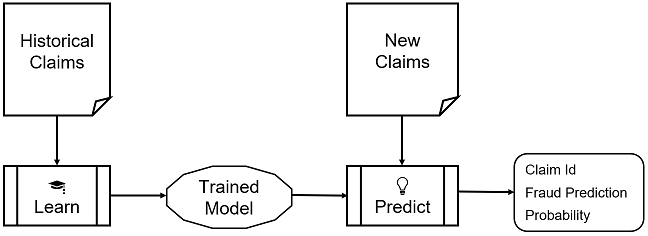Import the sample data included with the APL package to SAP HANA database.

```# Connect using the HANA secure user store
from hana_ml import dataframe as hd
conn = hd.ConnectionContext(userkey='MLMDA_KEY')
sql_cmd = 'SELECT * FROM "APL_SAMPLES"."AUTO_CLAIMS_FRAUD" ORDER BY CLAIM_ID'
hdf_train = hd.DataFrame(conn, sql_cmd)
```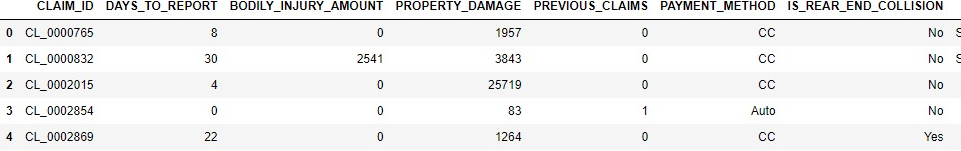Train a classification model on historical claims data with known fraud and display performance metrics of the trained model.

```# Create and train model
apl_model.fit(hdf_train, label='IS_FRAUD', key='CLAIM_ID')

print('\x1b[1m'+ 'MODEL PERFORMANCE' + '\x1b[0m')
sql_where = "\"Partition\"='Validation' and \"Indicator\" in ('AUC','Classification Rate')"
df = apl_model.get_debrief_report('ClassificationRegression_Performance').filter(sql_where).collect()
df = df[['Partition','Indicator','Value']]
df
```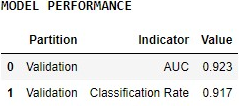Check how each variable contributes to the prediction.

```print('\x1b[1m'+ 'VARIABLES IMPORTANCE' + '\x1b[0m')
df = apl_model.get_debrief_report('ClassificationRegression_VariablesContribution').collect()
df = df.drop(columns=['Oid', 'Rank','Method'])
df = df[(df['Contribution'] > 0)]
format_dict = {'Contribution':'{0:,.2%}','Cumulative':'{0:,.2%}'}
df.sort_values(by=['Contribution'], ascending=False).style.format(format_dict).hide_index()
```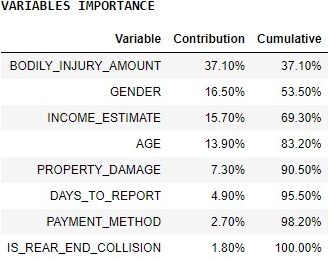Alternatively, see the contributions on a bar chart.

```import matplotlib.pyplot as plt
c_title = "Relative Contribution"
df = df.sort_values(by=['Contribution'], ascending=True)
df.plot(kind='barh', x='Variable', y='Contribution', title=c_title, legend=False, fontsize=12)
plt.show()
```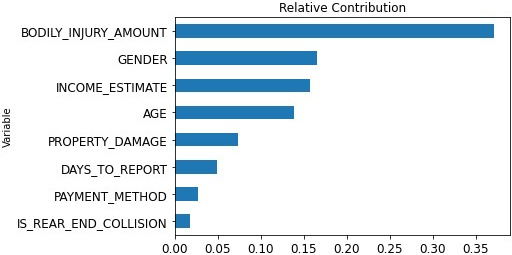Predict if each new claim is fraudulent or not.

```hdf_apply = conn.table('AUTO_CLAIMS_NEW', schema='APL_SAMPLES')
df = apl_model.predict(hdf_apply).collect()
```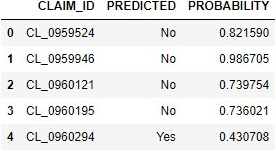## Visualizers Module

hana-ml provides various functions to visualize the dataset and model. The list is shown below:

An UnifiedReport example is shown below.

```# Import required module
from hana_ml.algorithms.pal.model_selection import GridSearchCV
from hana_ml.algorithms.pal.model_selection import RandomSearchCV

# Create a UnifiedClassification object
gscv = GridSearchCV(estimator=hgc,
param_grid={'learning_rate': [0.1, 0.4, 0.7, 1],
'n_estimators': [4, 6, 8, 10],
'split_threshold': [0.1, 0.4, 0.7, 1]},
train_control=dict(fold_num=5,
resampling_method='cv',
random_state=1,
ref_metric=['auc']),
scoring='error_rate')
gscv.fit(data=diabetes_train,
key='ID',
label='CLASS',
partition_method='stratified',
partition_random_state=1,
stratified_column='CLASS',
build_report=True)
```

To look at the dataset report.

```UnifiedReport(diabetes_train).build().display()
```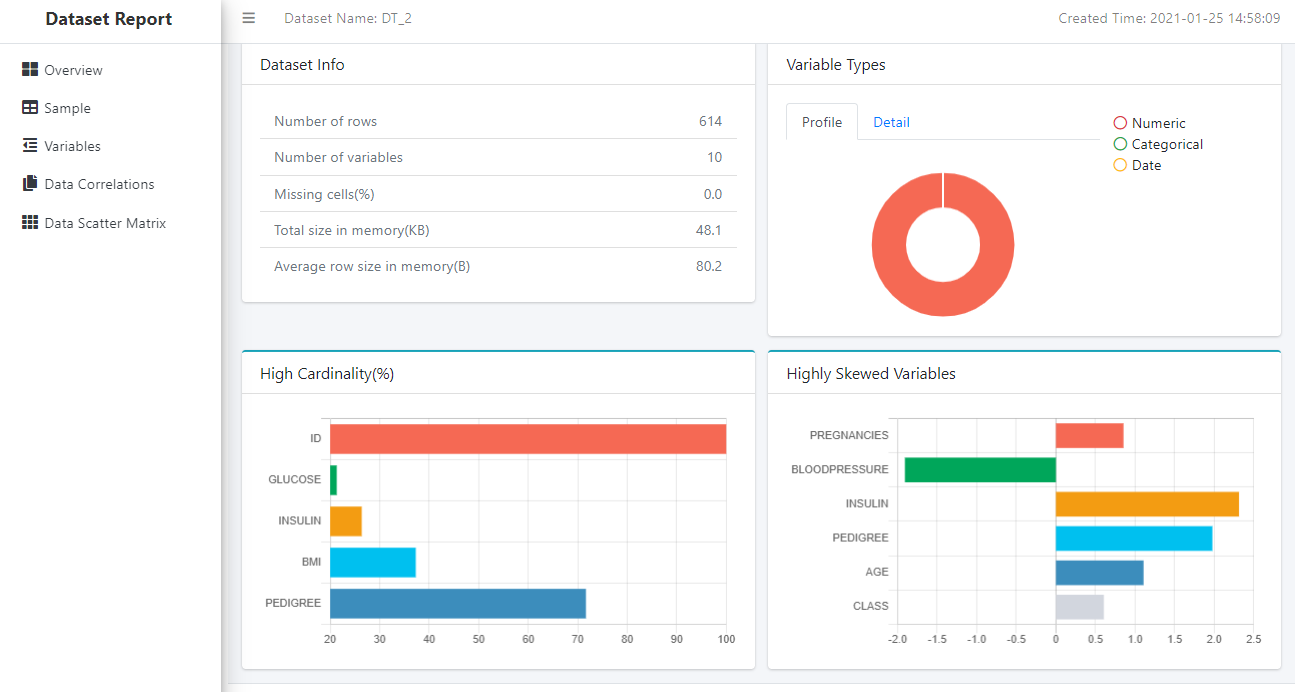To see the model report.

```UnifiedReport(gscv.estimator).display()
```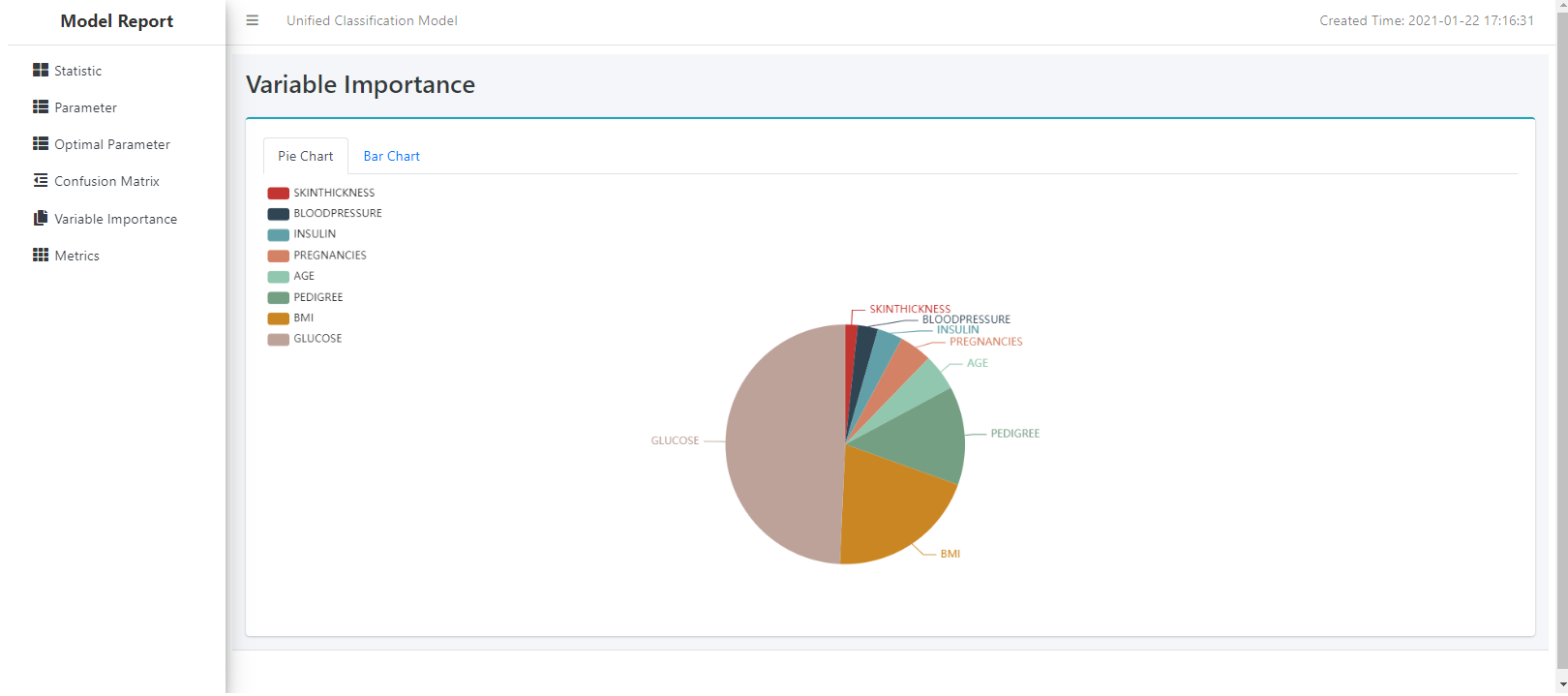## Spatial and Graph Features

Python machine learning client for SAP HANA introduces additional engines that can be used for analytics focused on Geospatial and Graph or network modeled data.

The Geospatial features contain integration of these file types through the create_dataframe functions:

• create_data_frame_from_shapefile (Sourced from shape files including dbf, shp, or zip formats).

• create_data_frame_from_pandas (Sourced from csv with a given geometry column and spatial reference id).

The Graph features contain integration of the same file types as well as making use of existing graph workspaces.

The hana_ml.graph package contains the following ways to create a Graph object representation:

• create_graph_from_dataframes (Sourced from csv pairs of vertices and edges).

• create_graph_from_hana_dataframes (Sourced from SAP HANA dataframes).

• Graph() (Main object created based on an existing graph workspace)

With the following methods the graph objects on the database can be discovered:

• discover_graph_workspaces (Metadata of all workspaces)

• discover_graph_workspace (Metadata of a specific workspace

Once a Graph object is created, it functions in a similar manner as the PAL and APL libraries which return Pandas Dataframes for the user. In the case of SAP HANA Graph the following functions are currently offered.

• edges (Pandas Dataframe of the edge table or links in other terms).

• vertices (Pandas Dataframe of the vertex table or nodes in other terms).

• has_vertices (Check if vertices exist in a graph)

• source (Find a source vertex for an edge)

• target (Find a target vertex for an edge)

• drop (Delete a graph workspace)

The hana_ml.graph.algorithms package contains a list of algorithms that can be applied to an graph object:

• Neighbors (Pandas Dataframe of the vertices that are connected to a given vertex key).

• NeighborsSubgraph (Pandas Dataframe of the vertices that are connected to a given vertex key and a Pandas Dataframe of the edges included).

• ShortestPath (Pandas Dataframe of the vertices that are on the shortest path between source and target vertex keys and a Pandas Dataframe of the edges that make the path).

• KShortestPath (Top-k shortest paths)

## Summary

Python machine learning client for SAP HANA (hana-ml) provides a set of Python APIs and functions for creating and manipulating SAP HANA DataFrames, training and scoring Machine Learning models. These functions ensure that the model training and prediction executes directly in SAP HANA. This offers better performance by executing close to the data, while ensuring there is no data transfer between the client and the server.